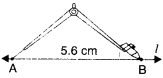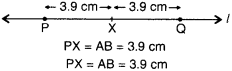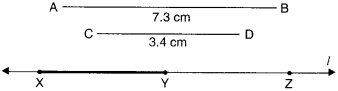NCERT Solutions for Class 6 Maths Chapter 14 Practical Geometry Ex 14.2 are part of NCERT Solutions for Class 6 Maths. Here we have given NCERT Solutions for Class 6 Maths Chapter 14 Practical Geometry Ex 14.2.

 Board CBSE Textbook NCERT Class Class 6 Subject Maths Chapter Chapter 14 Chapter Name Practical Geometry Exercise Ex 14.2 Number of Questions Solved 5 Category NCERT Solutions

## NCERT Solutions for Class 6 Maths Chapter 14 Practical Geometry Ex 14.2

Question 1.
Draw a line segment of length 7.3 cm. using a ruler.
Solution :
Using ruler, we mark two points A and B which are 7.3 cm apart. Join A and B and get AB. $$\overline { AB }$$ is a line segment of length 7.3 cm.Question 2.
Construct a line segment of length 5.6 cm using ruler and compasses.Solution :
Step 1. Draw a line l. Mak a point A on line l.
Step 2. Place the compasses pointer on the ∠ero mark on the ruler. Open it to place the pencil point upto the 5.6 cm mark.
Step 3. Without changing the opening of the compasses, place the pointer on A and swing an arc to cut / at B.
Step 4. AB is a line segment of required length.

Question 3.
Construct $$\overline { AB }$$ of length 7.8 cm. From this cut off $$\overline { AC }$$ of length 4.7 cm. Measure $$\overline { BC }$$.
Solution :
Steps of Construction
Step 1. Draw a line l. Mark a point A on line l.
Step 2. Place the compasses pointer on the ∠ero mark on the ruler. Open it to place the pencil point upto the 7.8 cm mark.Step 3. Without changing the opening of the compasses, place the pointer on A and swing an arc to cut / at B.
Step 4. $$\overline { AB }$$ is a line segment of length 7.8 cm.
Step 5. Place the compasses pointer on the ∠ero mark on the ruler. Open it to place the pencil point upto 4.7 cm mark.
Step 6. Without changing the opening of the compasses, place the pointer on A and swing an arc to cut / at C.
Step 7. $$\overline { AC }$$ is a line segment of length 4.7 cm. On measurement. $$\overline { BC }$$ =3.1 cm.

Question 4.
Given $$\overline { AB }$$ of length 3.9 cm, construct $$\overline { PQ }$$ such that the length of $$\overline { PQ }$$ is twice that of AB Verify by measurement.(Hint : Construct $$\overline { PX }$$ such that length of PX = length of $$\overline { AB }$$; then cut off $$\overline { XQ }$$ such that $$\overline { XQ }$$ also has the length of $$\overline { AB }$$).
Solution :
Steps of Construction
Step 1. Draw a line I. Mark a point P on line l.
Step 2. Place the compasses pointer on the A mark of the given line segment $$\overline { AB }$$ . Open it to place the pencil point upto B mark of the given line segment $$\overline { AB }$$.Step 3. Without changing the opening of the compasses, place the pointer on P and swing an arc to cut l at X.
Step 4. Again without changing the opening of the compasses place the compasses pointer on the X mark of line / and swing an arc to cut / at Q.
Step 5. $$\overline { PQ }$$ is a line segment of length twice that of $$\overline { AB }$$. Please verify yourself by measurement.

Question 5.
Given $$\overline { AB }$$ of length 7.3 cm and $$\overline { CD }$$ of length 3.4 cm, construct a line segment $$\overline { XY }$$ such that the length of $$\overline { XY }$$ is equal to the difference between the lengths of $$\overline { AB }$$ and $$\overline { CD }$$ . Verify by measurement.
Solution :
Steps of Construction
Step 1. Draw a line l. Mark a point X on line l.
Step 2. Place the compasses pointer on the A mark of the given line segment $$\overline { AB }$$. Open it to place the pencil point upto B mark of the given line segment $$\overline { AB }$$.
Step 3. Without changing the opening of the compasses, place the pointer of compasses on X and swing an arc to cut / at ∠.
Step 4. Place the compasses pointer on the C mark of the given line segment $$\overline { CD }$$. Open it to place the pencil point upto D mark of the given line segment $$\overline { CD }$$.
Step 5. Without changing the opening of the compasses, place the pointer of compasses on ∠ and swing an arc towards X to cut l at Y.
Step 6. $$\overline { XY }$$ is a required line segment of length = the difference between the lengths of $$\overline { AB }$$ and $$\overline { CD }$$.